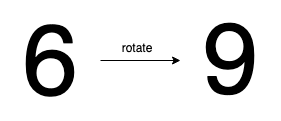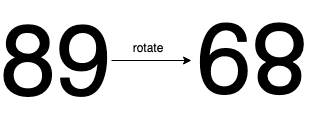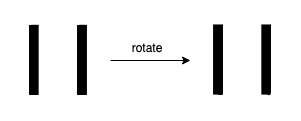## 1056. Confusing Number

Given a number `N`, return `true` if and only if it is a confusing number, which satisfies the following condition:

We can rotate digits by 180 degrees to form new digits. When 0, 1, 6, 8, 9 are rotated 180 degrees, they become 0, 1, 9, 8, 6 respectively. When 2, 3, 4, 5 and 7 are rotated 180 degrees, they become invalid. A confusing number is a number that when rotated 180 degrees becomes a different number with each digit valid.

Example 1:```Input: 6
Output: true
Explanation:
We get `9` after rotating `6`, `9` is a valid number and `9!=6`.
```

Example 2:```Input: 89
Output: true
Explanation:
We get `68` after rotating `89`, `86` is a valid number and `86!=89`.
```

Example 3:```Input: 11
Output: false
Explanation:
We get `11` after rotating `11`, `11` is a valid number but the value remains the same, thus `11` is not a confusing number.
```

Example 4:```Input: 25
Output: false
Explanation:
We get an invalid number after rotating `25`.
```

Note:

1. `0 <= N <= 10^9`
2. After the rotation we can ignore leading zeros, for example if after rotation we have `0008` then this number is considered as just `8`.

## Rust Solution

``````struct Solution;

impl Solution {
fn rotate(d: i32) -> Option<i32> {
match d {
0 => Some(0),
1 => Some(1),
6 => Some(9),
8 => Some(8),
9 => Some(6),
_ => None,
}
}

fn confusing_number(mut n: i32) -> bool {
let num = n;
let mut rev = 0;
while n > 0 {
let d = n % 10;
if let Some(t) = Self::rotate(d) {
rev *= 10;
rev += t;
n /= 10;
} else {
return false;
}
}
rev != num
}
}

#[test]
fn test() {
assert_eq!(Solution::confusing_number(6), true);
assert_eq!(Solution::confusing_number(89), true);
assert_eq!(Solution::confusing_number(11), false);
assert_eq!(Solution::confusing_number(25), false);
}
``````

Having problems with this solution? Click here to submit an issue on github.